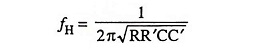## Active Filters Interview Questions and Answers:

1. What is meant by ‘frequency response’ of a filter?

Ans. A graph of the magnitude of the gain versus frequency is called a frequency response plot.

2. The gain of a filter is a complex number. What is meant by it?

Ans. Because at a given frequency, the gain has a specific magnitude as well as a phase angle, the gain of a filter is said to be a complex number.

3. What is meant by -3 dB frequency?

Ans. -3 dB frequency is one at which the power is reduced to one half of the maximum magnitude.

4. What are the commonly used filters?

Ans. The most commonly used filters are low-pass, high-pass, band-pass, band-reject and all-pass filters.

5. Distinguish between active and passive filters.

Ans. A passive filter is formed with passive components, such as resistors, inductors and capacitors while active filters make use of transistors or op-amps in addition to resistors and capacitors.

6. Define filter parameters.

Ans. The filter exhibits a wide variety of parameters such as order of filter, cutoff frequencies i.e., upper cutoff frequency and lower cutoff frequency, passband gain, break frequency etc.

7. Explain why active filters are preferred over passive filters?

Ans. Active filters are preferred over passive filters because they provide gain and frequency adjustment flexibility. They pose no loading problem because of high input impedance and low output impedance and they are comparatively less expensive.

8. What is a low-pass filter?

Ans. A filter that provides a constant output from dc up to a cutoff frequency fc and then passes no signal above that frequency is called a low-pass filter.

9. Define first-order low-pass filter? What does order of a filter signifies?

Ans. A first order low-pass filter is that in which there is a constant gain from 0 Hz to a high cutoff frequency fH and beyond fH the gain decreases at constant rate with the increase in frequency. The rate at which the gain rolls off beyond fH is 20 dB/decade or 6 dB/octave.

The order of a filter signifies that at what rate stopband response increases or decreases. Higher the order of the filter, better are the attenuation characteristics of the filter. It means that as the order of the filter is increased, the actual stopband response of the filter approaches its ideal stopband characteristics.

10. Define second-order low-pass filter? What does order of a filter signify?

Ans. A second-order low-pass filter is that in which there is a constant gain from 0 Hz to a high cutoff frequency fH and beyond fH the gain decreases at constant rate with the increase in frequency. The rate at which the gain rolls off beyond fH is 40 dB/decade.

The order of a filter signifies that at what rate stop band response increases or decreases. Higher the order of the filter, better are the attenuation characteristics of the filter. It means that as the order of the filter is increased, the actual stopband response of the filter approaches its ideal stopband characteristics.

11. Give expression for the upper cutoff frequency of a second-order low-pass filter.

Ans. The upper cutoff frequency of a second-order low-pass filter is given by the expression12. What is a high-pass filter?

Ans. A filter that provides or passes signals above a cutoff frequency fis called a high-pass filter. It has a zero gain starting from zero to a frequency fc and above this frequency the gain is constant.

13. What is the advantage of increasing the order of a filter?

Ans. With increasing the order of a filter, the actual stopband response of the filter approaches its ideal stopband characteristics.

14. How does the shape of the frequency response plots near the critical frequency depend upon the damping coefficient?

Ans. The Butterworth filter (α = 1.414) offers optimum flatness. Increase in damping coefficient causes considerable passband attenuation and slow initial roll-off, but assures good transient, and time delay response. Decrease in damping coefficient (Chebyshev) allows much faster initial roll-off, but introduces passband ripple and poor transient response.

15. Why do we use higher order filters?

Ans. Higher order filters are used to obtain stopband response of the filter approaching ideal stopband characteristics.

16. What is the wide bandpass filter?

Ans. A wide bandpass filter has a wide passband between two cutoff frequencies fH and fL where fH >> fL and two stopbands at 0 < f < fand f  > fH.

17. What is a notch filter?

Ans. Notch filter is a narrow band-stop filter. It notches out or blocks frequencies near centre frequency fc, also called a notch-out frequency.

18. What is a state variable filter?

Ans. The state-variable filter, also called a universal filter, offers several special features not available with the other simpler filters. It makes use of three or four op-amps and two feedback paths.

Scroll to Top